# How to Calculate and Solve for Quantity of Soil Moved | Water Budget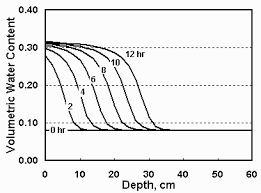The image above represents quantity of soil moved.

To compute for quantity of soil moved, two essential parameters are needed and these parameters are Excess Wind Velocity (vw) and Particle Diameter (dp).

The formula for calculating quantity of soil moved:

Q = vw³ . dp

Where;

Q = Quantity of Soil Moved
vw = Excess Wind Velocity
dp = Particle Diameter

Let’s solve an example;
Find the quantity of soil moved when the excess wind velocity is 12 and the particle diameter is 20.

This implies that;

vw = Excess Wind Velocity = 12
dp = Particle Diameter = 20

Q = vw³ . dp
Q = (12)³ . (20)
Q = (1728) . (20)
Q = 34560

Therefore, the quantity of soil moved is 34560.

Calculating the Excess Wind Velocity when the Quantity of Soil Moved and the Particle Diameter is Given.

vw³ = 3Q / dp

Where;

vw = Excess Wind Velocity
Q = Quantity of Soil Moved
dp = Particle Diameter

Let’s solve an example;
Find the excess wind velocity when the quantity of soil moved is 30 and the particle diameter is 10.

This implies that;

Q = Quantity of Soil Moved = 30
dp = Particle Diameter = 10

vw³ = 3Q / dp
vw³ = 330 / 10
vw³ = 3√3
vw³ = 1.442

Therefore, the excess wind velocity is 1.442.

Calculating the Particle Diameter when the Quantity of Soil Moved and the Excess Wind Velocity is Given.

dp = Q / vw³

Where;

dp = Particle Diameter
Q = Quantity of Soil Moved
vw = Excess Wind Velocity

Let’s solve an example;
Find the particle diameter when the excess wind velocity is 5 and the quantity of soil moved is 40.

This implies that;

Q = Quantity of Soil Moved = 40
vw = Excess Wind Velocity = 5

dp = Q / vw³
dp = 40 /
dp = 40 / 125
dp = 0.32

Therefore, the particle diameter is 0.32.

Nickzom Calculator – The Calculator Encyclopedia is capable of calculating the quantity of soil moved.

To get the answer and workings of the quantity of soil moved using the Nickzom Calculator – The Calculator Encyclopedia. First, you need to obtain the app.

You can get this app via any of these means:

To get access to the professional version via web, you need to register and subscribe for NGN 1,500 per annum to have utter access to all functionalities.
You can also try the demo version via https://www.nickzom.org/calculator

Apple (Paid) – https://itunes.apple.com/us/app/nickzom-calculator/id1331162702?mt=8
Once, you have obtained the calculator encyclopedia app, proceed to the Calculator Map, then click on Agricultural under Engineering.Now, Click on Water Budget under AgriculturalNow, Click on Quantity of Soil Moved under Water Budget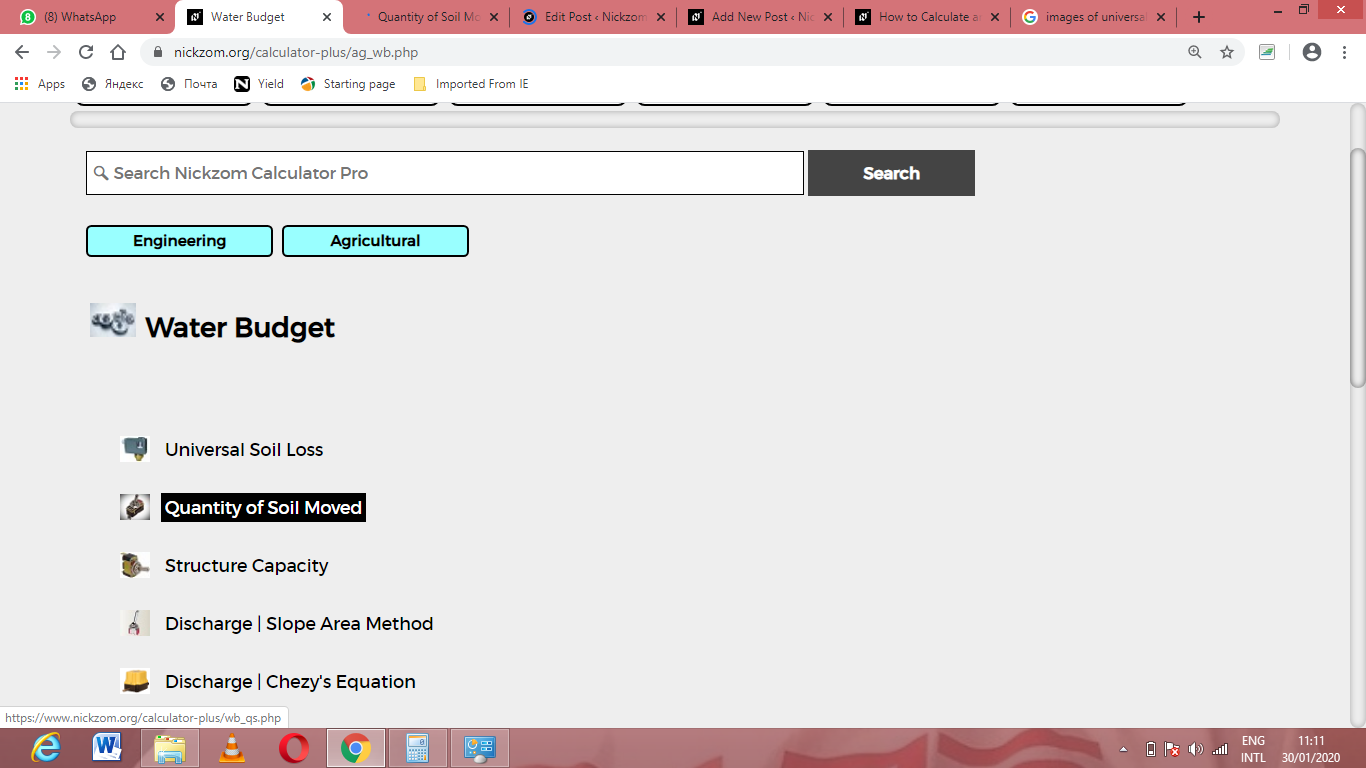The screenshot below displays the page or activity to enter your values, to get the answer for the quantity of soil moved according to the respective parameters which are the Excess Wind Velocity (vw) and Particle Diameter (dp).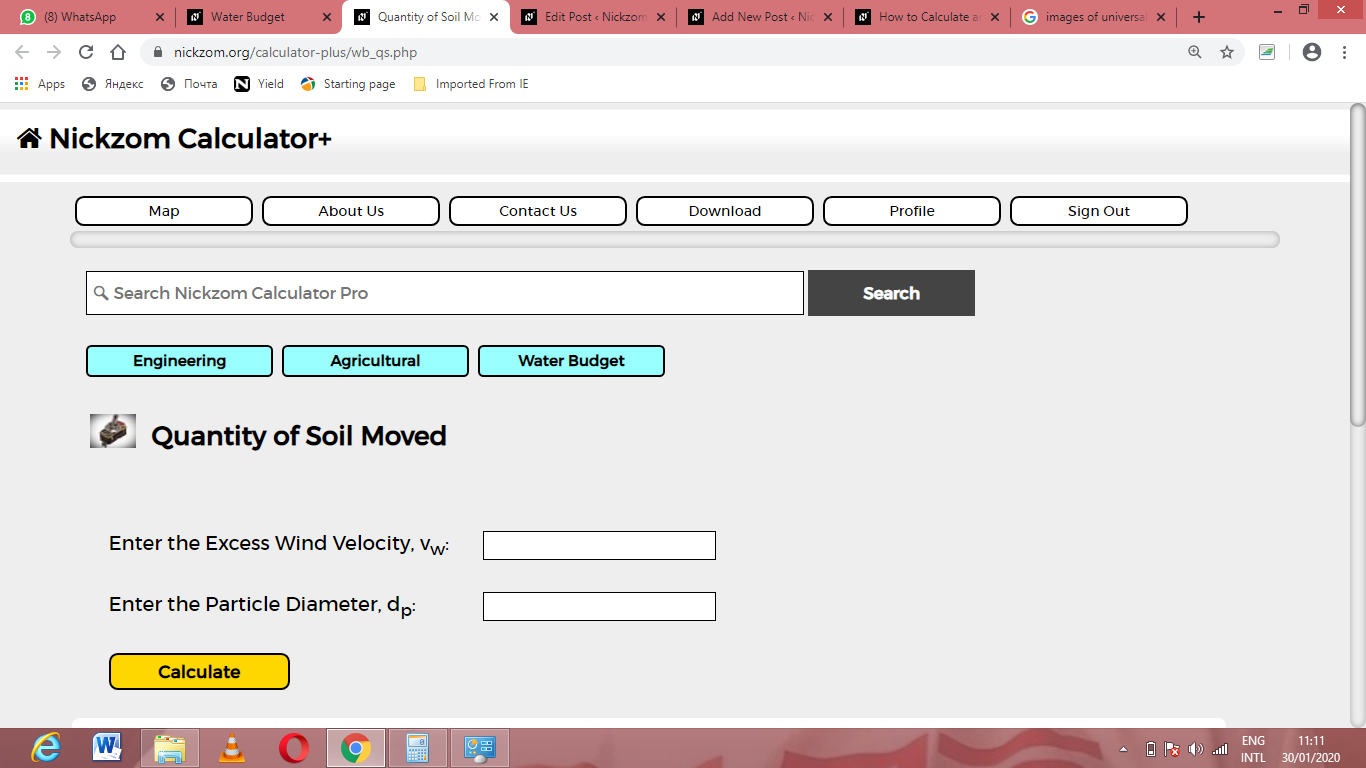Now, enter the values appropriately and accordingly for the parameters as required by the Excess Wind Velocity (vw) is 12 and Particle Diameter (dp) is 20.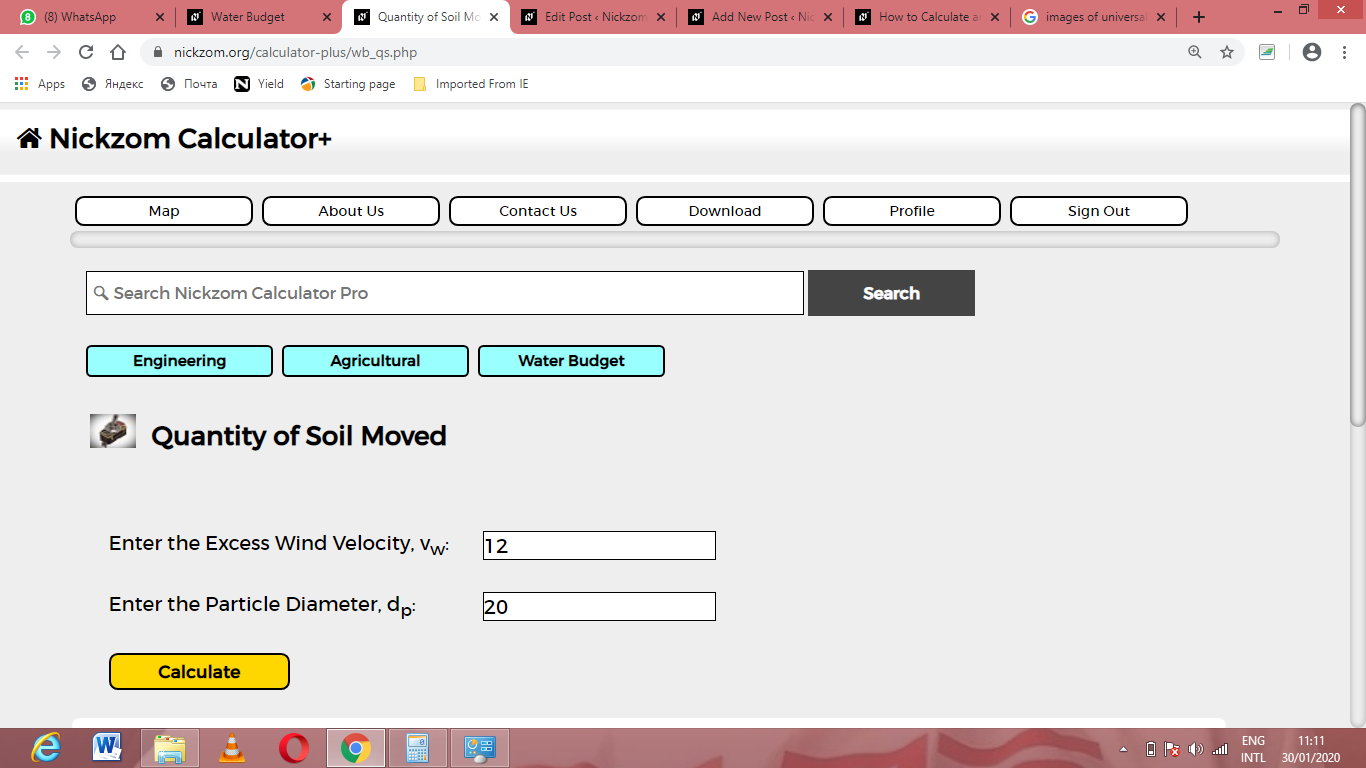Finally, Click on Calculate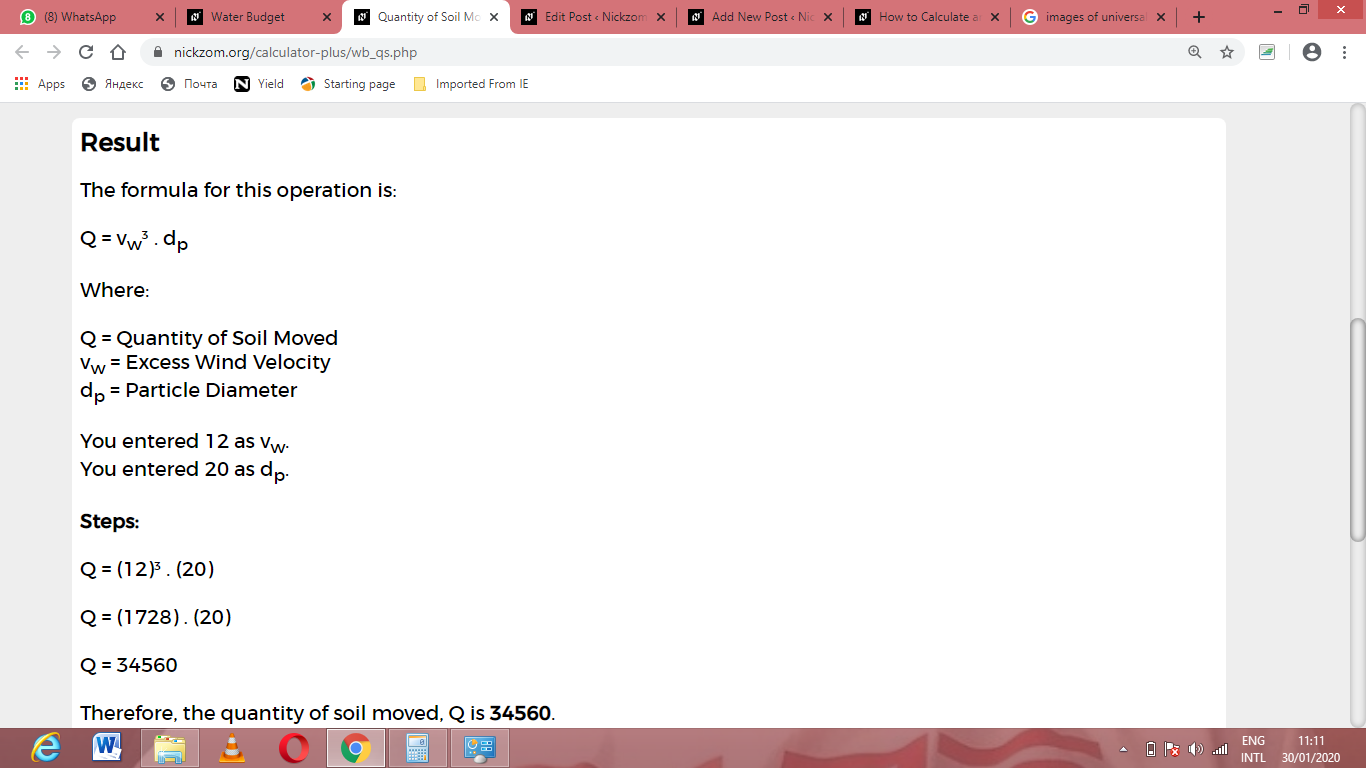As you can see from the screenshot above, Nickzom Calculator– The Calculator Encyclopedia solves for the quantity of soil moved and presents the formula, workings and steps too.